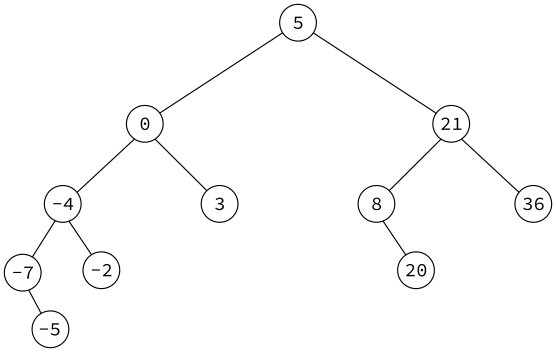A document from MCS 275 Spring 2022, instructor David Dumas. You can also get the notebook file.

# MCS 275 Spring 2022 Homework 8 Solutions¶

• Course Instructor: David Dumas
• Solutions prepared by: Johnny Joyce

## Instructions:¶

• Complete the problems below, which ask you to write Python scripts.
• Upload your python code directly to gradescope, i.e. upload the .py files containing your work. (If you upload a screenshot or other file format, you won't get credit.)

This homework assignment must be submitted in Gradescope by Noon central time on Tuesday 8 March 2022.

### Collaboration¶

Collaboration is prohibited, and you may only access resources (books, online, etc.) listed below.

### Resources you may consult¶

The course materials you may refer to for this homework are:

### Point distribution¶

This homework assignment has two problems, numbered 2 and 3. The grading breakdown is:

Points Item
4 Problem 2
4 Problem 3
10 Total

The part marked "autograder" reflects points assigned to your submission based on some simple automated checks for Python syntax, etc.. The result of these checks is shown immediately after you submit.

### What to do if you're stuck¶

Ask your instructor or TA a question by email, in office hours, or on discord.

## Problem 1 doesn't exist¶

In Gradescope, the score assigned to your homework submission by the autograder (checking for syntax and docstrings) will be recorded as "Problem 1". Therefore, the numbering of the actual problems begins with 2.

## Prepare¶

Download trees.py from the course sample code repository and copy it to a new file hwk8.py. (It's also OK to take the modified version of trees.py you worked on in lab this week and copy it.) Both questions on this assignment ask you to make changes to this file.

## Problem 2: Leaf list¶

A node of a tree is called a leaf if it has no children.

Add a method leaves to class Node in hwk8.py that, when called on the root of a tree, will find all leaf nodes in that tree and return a list of them. (So the return value should be a list of Node objects.)

# Solution¶

Inside class Node:

In [ ]:
    def leaves(self):
'''Returns list of all leaf nodes'''
if self.left == None and self.right == None:
return [self] # If current node has no children, then it must be a leaf
elif self.left == None:
return self.right.leaves()
elif self.right == None:
return self.left.leaves()
else:
return self.left.leaves() + self.right.leaves() # + for list addition


Example of usage (in some other Python file):

In :
import treevis
import treeutil

T = treeutil.random_bst(nodes=5,seed=21)
treevis.treeprint(T)

print("Leaves of tree T:")
print(T.leaves())

      

     

   .   .

Leaves of tree T:
[, , ]

• Note that each element of the list is contained in a set of square brackets [] since they are Node objects

## Problem 3: Positive elements of BST¶

Add a method positive_keys to class BST in hwk8.py that, when called on the root of a binary search tree containing floats or integers, will return a list of all the positive keys that appear in the binary search tree.

• A correct solution that always visits every node in the tree will be worth 2 points, because visiting every node is often quite inefficient.
• For full credit, a solution must ignore parts of the tree that can't possibly contain positive keys. For example, in this binary tree:there is no need to explore the left subtree of the node with key 0, because all keys in that subtree are guaranteed to be less than or equal to 0 (i.e. NOT positive).

# Solution¶

Inside class BST:

In [ ]:
    def positive_keys(self):
'''Returns list of keys strictly greater than 0'''

# First case: If current key is negative (or 0), no need to look to the left
if self.key <= 0:
if self.right != None: # Even if key is negative, we might need to look to the right
return self.right.positive_keys()
else:
return [] # Case where no positive keys were found

# Second case: If key is positive, then keep current key and check both left and right sides
else:
L = [self.key]
if self.left != None:
L.extend(self.left.positive_keys())
if self.right != None:
L.extend(self.right.positive_keys())
return L


Testing the method:

In :
import trees
import treevis

# Build a tree with some arbitrary positive and negative values
T = trees.BST(10)
for k in [15, 4, -6, 13, 1, -3, 100, -50, 2, 11,12, 500]:
T.insert(k)

treevis.treeprint(T)

print("Positive keys of tree T:")
print(T.positive_keys())

                                      

                                     

[-6]                 .                                 

[-50]              .         .               .         .       

.    .   [-3]    .    .    .    .    .     .    .    .    .    .    .

Positive keys of tree T:
[10, 4, 1, 2, 15, 13, 11, 12, 100, 500]